# Hidden Markov Models HMMs Cheng Xiang Zhai Department

• Slides: 34Hidden Markov Models (HMMs) Cheng. Xiang Zhai Department of Computer Science University of Illinois, Urbana-Champaign 1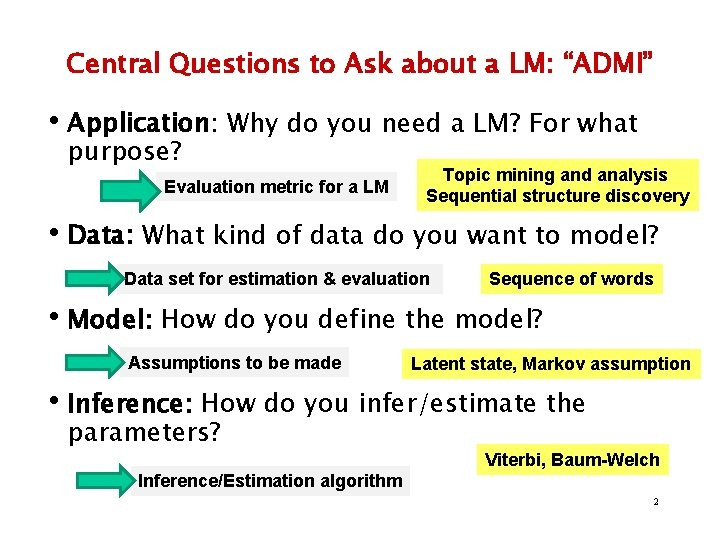Central Questions to Ask about a LM: “ADMI” • Application: Why do you need a LM? For what purpose? Evaluation metric for a LM Topic mining and analysis Sequential structure discovery • Data: What kind of data do you want to model? Data set for estimation & evaluation Sequence of words • Model: How do you define the model? Assumptions to be made Latent state, Markov assumption • Inference: How do you infer/estimate the parameters? Viterbi, Baum-Welch Inference/Estimation algorithm 2Modeling a Multi-Topic Document A document with 2 subtopics, thus 2 types of vocabulary … text mining passage How do we model such a document? food nutrition passage How do we “generate” such a document? text mining passage How do we estimate our model? food nutrition passage … We’ve already seen one solution – unigram mixture model + EM This lecture is about another (better) solution – HMM + EM 3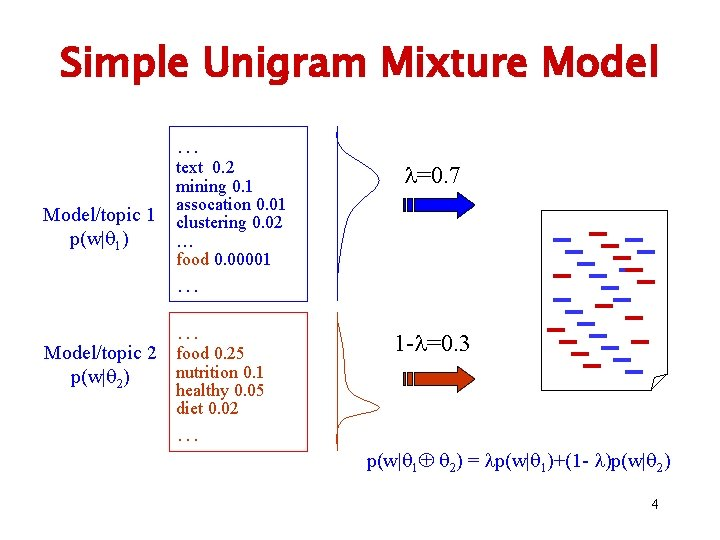Simple Unigram Mixture Model … Model/topic 1 p(w| 1) text 0. 2 mining 0. 1 assocation 0. 01 clustering 0. 02 … food 0. 00001 =0. 7 … … Model/topic 2 food 0. 25 nutrition 0. 1 p(w| 2) 1 - =0. 3 healthy 0. 05 diet 0. 02 … p(w| 1 2) = p(w| 1)+(1 - )p(w| 2) 4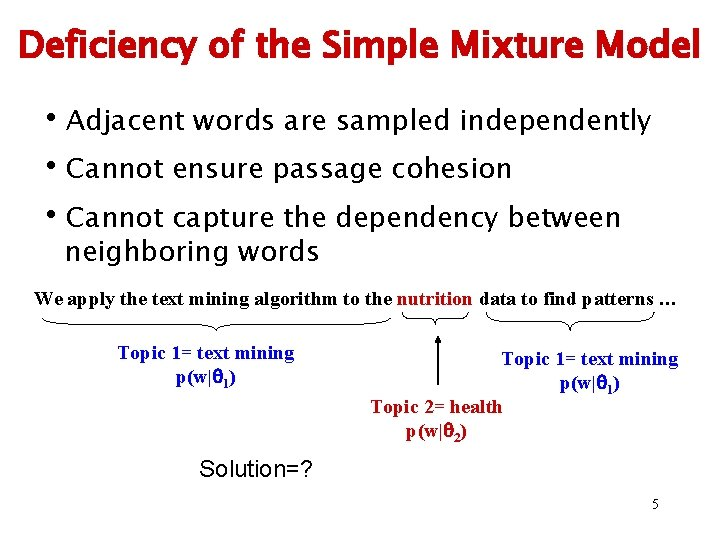Deficiency of the Simple Mixture Model • Adjacent words are sampled independently • Cannot ensure passage cohesion • Cannot capture the dependency between neighboring words We apply the text mining algorithm to the nutrition data to find patterns … Topic 1= text mining p(w| 1) Topic 2= health p(w| 2) Solution=? 5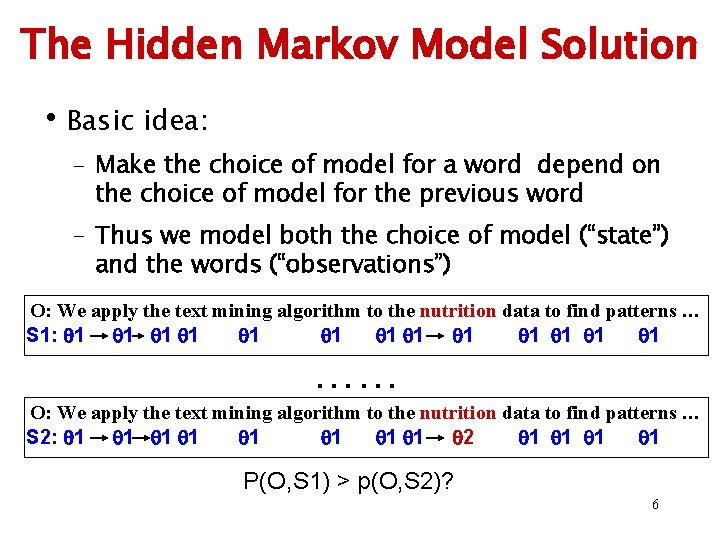The Hidden Markov Model Solution • Basic idea: – Make the choice of model for a word depend on the choice of model for the previous word – Thus we model both the choice of model (“state”) and the words (“observations”) O: We apply the text mining algorithm to the nutrition data to find patterns … S 1: 1 1 1 1 …… O: We apply the text mining algorithm to the nutrition data to find patterns … S 2: 1 1 1 1 2 1 1 P(O, S 1) > p(O, S 2)? 6Another Example: Passage Retrieval q Strategy I: Build passage index w Need pre-processing w Query independent boundaries Relevant w Efficient Passages (“T”) q Strategy II: Extract relevant passages dynamically w No pre-processing w Dynamically decide the boundaries Background text (“B”) w Slow O: We apply the text mining algorithm to the nutrition data to find patterns … S 1: B T T T BB T T O: …. . We apply the text mining … patterns. ……………… S 2: BB. . . BB T T T …. T BB…. . BBBB 7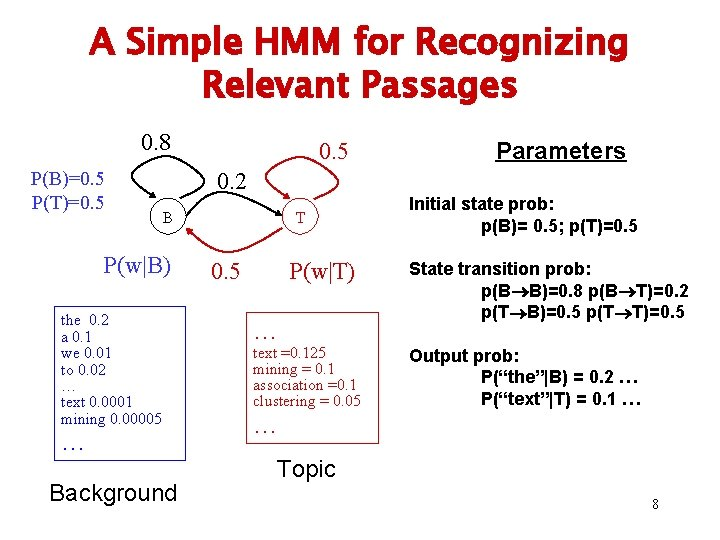A Simple HMM for Recognizing Relevant Passages 0. 8 P(B)=0. 5 P(T)=0. 5 Parameters 0. 2 B P(w|B) the 0. 2 a 0. 1 we 0. 01 to 0. 02 … text 0. 0001 mining 0. 00005 … Background T 0. 5 P(w|T) … text =0. 125 mining = 0. 1 association =0. 1 clustering = 0. 05 Initial state prob: p(B)= 0. 5; p(T)=0. 5 State transition prob: p(B B)=0. 8 p(B T)=0. 2 p(T B)=0. 5 p(T T)=0. 5 Output prob: P(“the”|B) = 0. 2 … P(“text”|T) = 0. 1 … … Topic 8A General Definition of HMM Initial state probability: N states State transition probability: M symbols Output probability: 9How to “Generate” Text? P(w|B) 0. 8 the 0. 2 a 0. 1 we 0. 01 is 0. 02 … method 0. 0001 mining 0. 00005 0. 2 P(B)=0. 5 B 0. 2 the … B … 0. 5 P(w|T) 0. 5 T 0. 5 0. 125 text T 0. 5 0. 1 text =0. 125 mining = 0. 1 algorithm =0. 1 clustering = 0. 05 T 0. 5 0. 1 … P(T)=0. 5 B 0. 8 B 0. 02 mining algorithm is 0. 2 0. 1 a T 0. 5 0. 05 B 0. 0001 clustering method P(BTT…, ”the text mining…”)=p(B)p(the|B) p(T|B)p(text|T) p(T|T)p(mining|T)… = 0. 5*0. 2 * 0. 2*0. 125 * 0. 5*0. 1… 10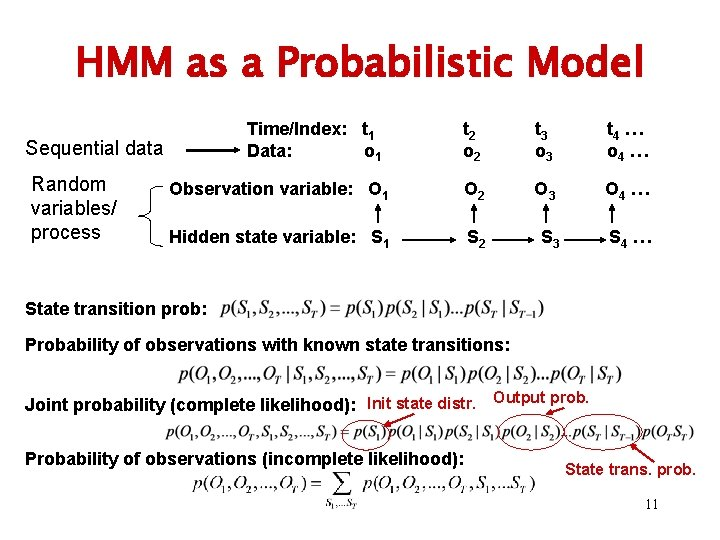HMM as a Probabilistic Model Time/Index: t 1 Data: o 1 t 2 o 2 t 3 o 3 t 4 … o 4 … Observation variable: O 1 O 2 O 3 O 4 … Hidden state variable: S 1 S 2 S 3 S 4 … Sequential data Random variables/ process State transition prob: Probability of observations with known state transitions: Joint probability (complete likelihood): Init state distr. Output prob. Probability of observations (incomplete likelihood): State trans. prob. 11Three Problems 1. Decoding – finding the most likely path Given: model, parameters, observations (data) Find: most likely states sequence (path) 2. Evaluation – computing observation likelihood Given: model, parameters, observations (data) Find: the likelihood to generate the data 12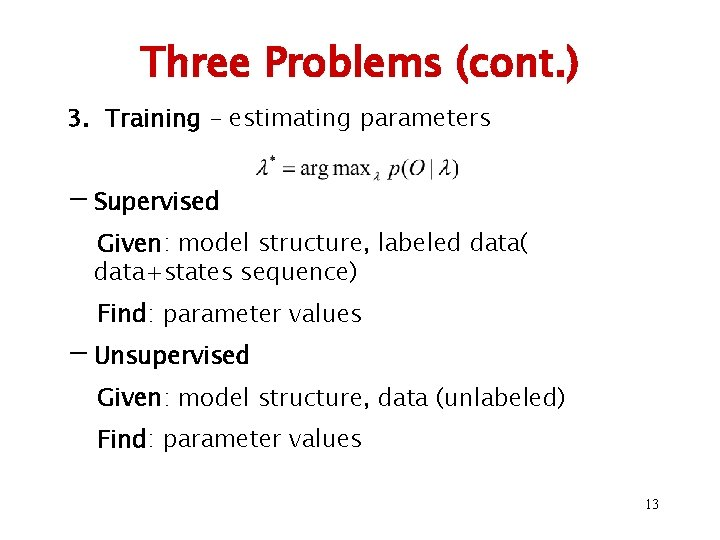Three Problems (cont. ) 3. Training – estimating parameters - Supervised Given: model structure, labeled data( data+states sequence) Find: parameter values - Unsupervised Given: model structure, data (unlabeled) Find: parameter values 13Problem I: Decoding/Parsing Finding the most likely path This is the most common way of using an HMM (e. g. , extraction, structure analysis)… 14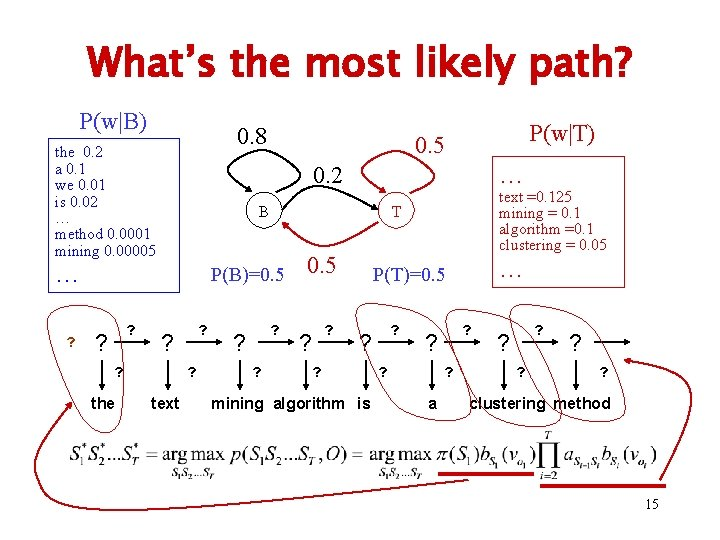What’s the most likely path? P(w|B) 0. 8 the 0. 2 a 0. 1 we 0. 01 is 0. 02 … method 0. 0001 mining 0. 00005 0. 2 ? ? ? the ? ? text ? ? ? text =0. 125 mining = 0. 1 algorithm =0. 1 clustering = 0. 05 T P(B)=0. 5 ? … B … ? P(w|T) 0. 5 ? ? … P(T)=0. 5 ? ? ? mining algorithm is ? ? a ? ? ? clustering method 15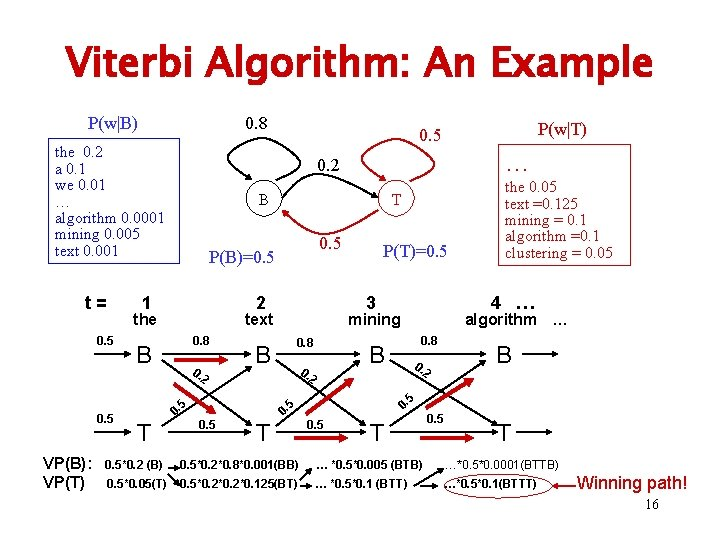Viterbi Algorithm: An Example P(w|B) 0. 8 the 0. 2 a 0. 1 we 0. 01 … algorithm 0. 0001 mining 0. 005 text 0. 001 t= B 0. 8 0. 2 P(T)=0. 5 3 text 0. 8 0. 2 2 T B 5 0. 0. 5 algorithm … B 0. the 0. 05 text =0. 125 mining = 0. 1 algorithm =0. 1 clustering = 0. 05 4 … mining B 5 VP(B): VP(T) 0. 5 2 B T T P(B)=0. 5 the 0. 5 … 0. 2 1 0. 5 P(w|T) 0. 5 T 0. 5*0. 2 (B) 0. 5*0. 2*0. 8*0. 001(BB) … *0. 5*0. 005 (BTB) …*0. 5*0. 0001(BTTB) 0. 5*0. 05(T) 0. 5*0. 2*0. 125(BT) … *0. 5*0. 1 (BTT) …*0. 5*0. 1(BTTT) Winning path! 16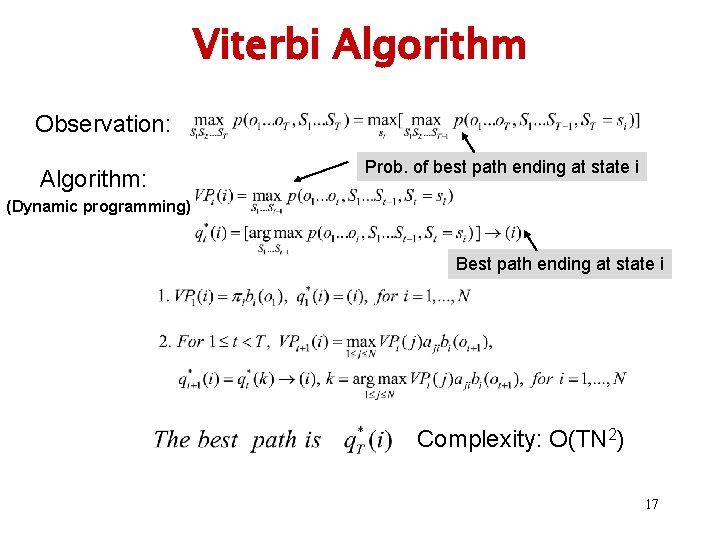Viterbi Algorithm Observation: Algorithm: Prob. of best path ending at state i (Dynamic programming) Best path ending at state i Complexity: O(TN 2) 17Problem II: Evaluation Computing the data likelihood § Another use of an HMM, e. g. , as a generative model for classification §Also related to Problem III – parameter estimation 18Data Likelihood: p(O| ) t= 0. 5 1 2 the text 0. 8 B 0. 2 In general, T 0. 8 0. algorithm … 0. 8 B 0. 2 2 T B 5 0. 0. 5 4 … mining B 5 0. 5 3 0. 5 T enumerate all paths BB… B BT… B TT… T Complexity of a naïve approach? 19The Forward Algorithm … … B B T T α 1 20The Forward Algorithm … … α 1 B B T T α 2 21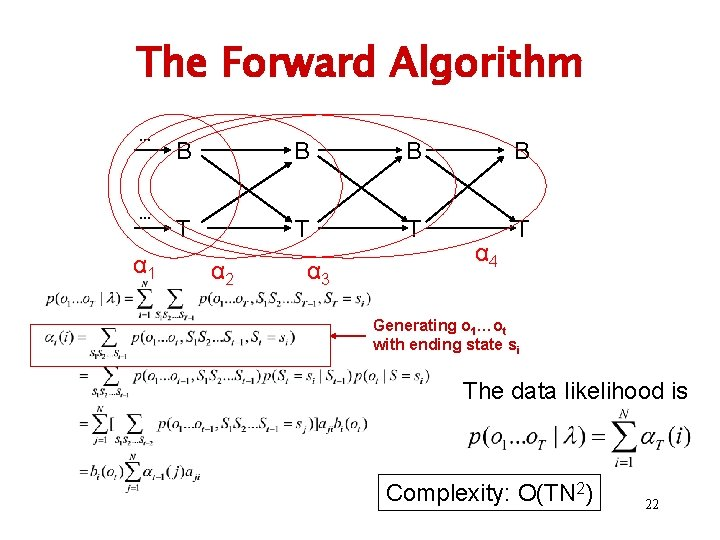The Forward Algorithm … … α 1 B B T T α 2 α 3 α 4 Generating o 1…ot with ending state si The data likelihood is Complexity: O(TN 2) 22Forward Algorithm: Example t= 0. 5 1 2 the text 0. 8 B 0. 2 0. 8 0. algorithm … 0. 8 B 0. 2 2 0. 5 T B 5 0. 0. T 4 … mining B 5 0. 5 3 0. 5 T 0. 5 1(B): 0. 5*p(“the”|B) 2(B): [ 1(B)*0. 8+ 1(T)*0. 5]*p(“text”|B) …… 1(T): 0. 5*p(“the”|T) 2(T): [ 1(B)*0. 2+ 1(T)*0. 5]*p(“text”|T) …… T P(“the text mining algorithm”) = 4(B)+ 4(T) 23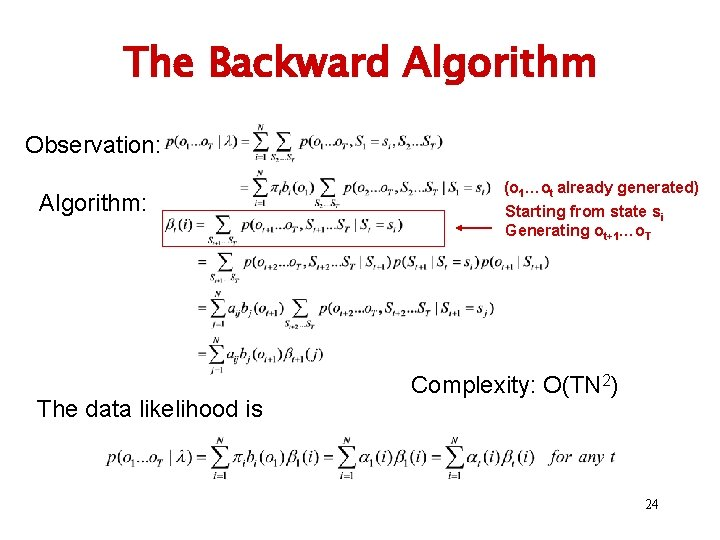The Backward Algorithm Observation: Algorithm: The data likelihood is (o 1…ot already generated) Starting from state si Generating ot+1…o. T Complexity: O(TN 2) 24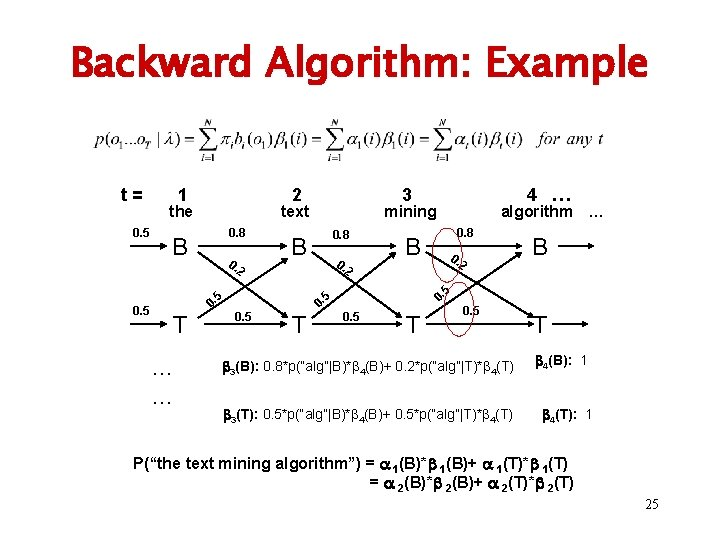Backward Algorithm: Example t= 0. 5 1 2 the text 0. 8 B 0. 2 T … … 0. 8 0. algorithm … 0. 8 B 0. 2 2 T B 5 0. 0. 5 4 … mining B 5 0. 5 3 0. 5 T 0. 5 3(B): 0. 8*p(“alg”|B)* 4(B)+ 0. 2*p(“alg”|T)* 4(T) 3(T): 0. 5*p(“alg”|B)* 4(B)+ 0. 5*p(“alg”|T)* 4(T) T 4(B): 1 4(T): 1 P(“the text mining algorithm”) = 1(B)* 1(B)+ 1(T)* 1(T) = 2(B)* 2(B)+ 2(T)* 2(T) 25Problem III: Training Estimating Parameters Where do we get the probability values for all parameters? Supervised vs. Unsupervised 26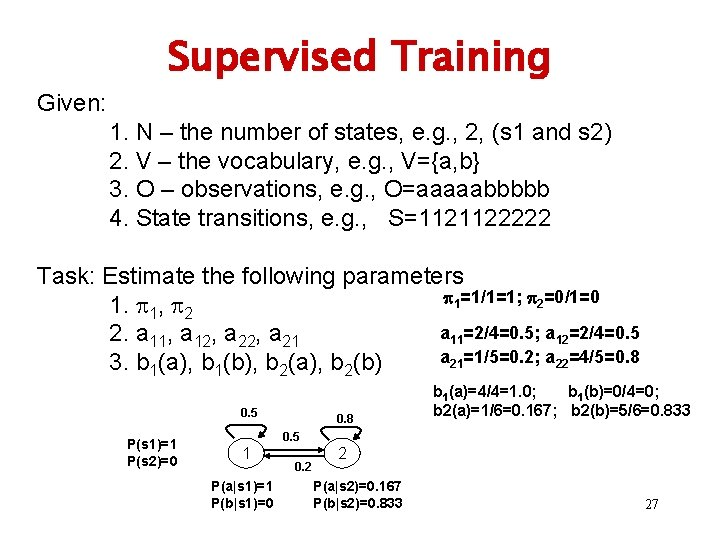Supervised Training Given: 1. N – the number of states, e. g. , 2, (s 1 and s 2) 2. V – the vocabulary, e. g. , V={a, b} 3. O – observations, e. g. , O=aaaaabbbbb 4. State transitions, e. g. , S=1121122222 Task: Estimate the following parameters 1=1/1=1; 2=0/1=0 1. 1, 2 a 11=2/4=0. 5; a 12=2/4=0. 5 2. a 11, a 12, a 21=1/5=0. 2; a 22=4/5=0. 8 3. b 1(a), b 1(b), b 2(a), b 2(b) 0. 5 P(s 1)=1 P(s 2)=0 0. 8 b 1(a)=4/4=1. 0; b 1(b)=0/4=0; b 2(a)=1/6=0. 167; b 2(b)=5/6=0. 833 0. 5 1 P(a|s 1)=1 P(b|s 1)=0 0. 2 2 P(a|s 2)=0. 167 P(b|s 2)=0. 833 27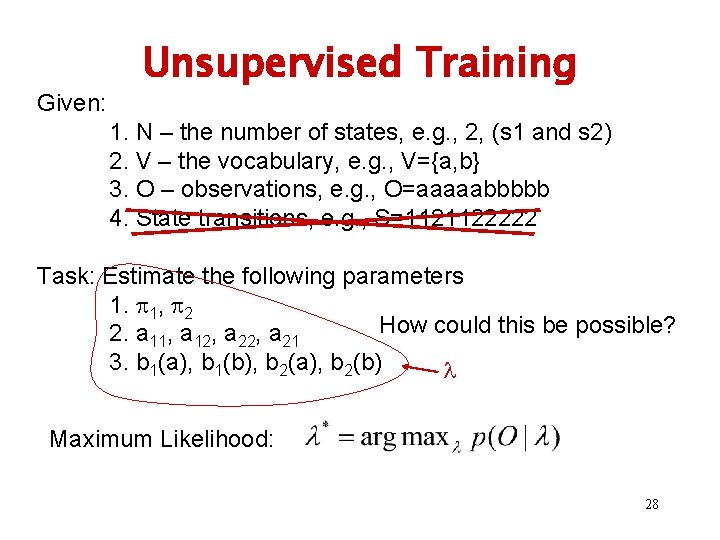Given: Unsupervised Training 1. N – the number of states, e. g. , 2, (s 1 and s 2) 2. V – the vocabulary, e. g. , V={a, b} 3. O – observations, e. g. , O=aaaaabbbbb 4. State transitions, e. g. , S=1121122222 Task: Estimate the following parameters 1. 1, 2 How could this be possible? 2. a 11, a 12, a 21 3. b 1(a), b 1(b), b 2(a), b 2(b) Maximum Likelihood: 28Intuition O=aaaaabbbbb, P(O, q 1| ) q 1=11111 P(O, q 2| ) P(O, q. K| ) q 2=11111112211 … q. K=22222 New ’ Computation of P(O, qk| ) is expensive … 29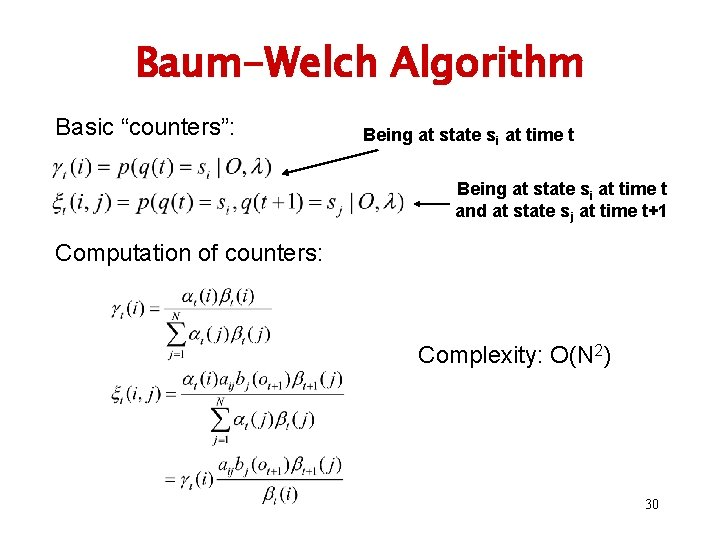Baum-Welch Algorithm Basic “counters”: Being at state si at time t and at state sj at time t+1 Computation of counters: Complexity: O(N 2) 30Baum-Welch Algorithm (cont. ) Updating formulas: Overall complexity for each iteration: O(TN 2) 31An HMM for Information Extraction (Research Paper Headers) 32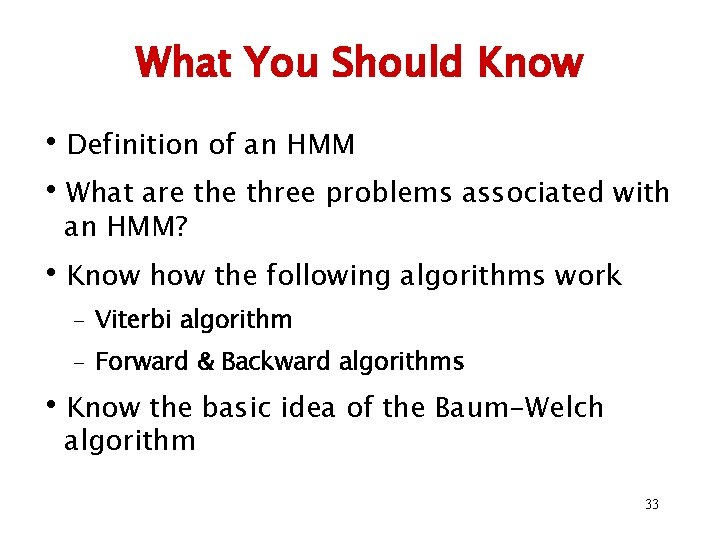What You Should Know • Definition of an HMM • What are three problems associated with an HMM? • Know how the following algorithms work – Viterbi algorithm – Forward & Backward algorithms • Know the basic idea of the Baum-Welch algorithm 33